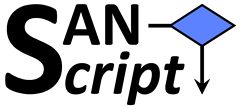# The SANscript LanguageSANscript (“Scientific Algorithm Notation Script”) is an interpreted programming language for scientific purposes which I am currently developing. It is already in use in the ORCA molecular dynamics module – the input language used there is SANscript. The language mixes elements from several other programming languages and is very permissive with regard to the input. Line breaks and commas (e.g. when separating function arguments) are only decorative and can be left out. Every SANscript input can be written in one single line if desired. The language is not case-sensitive. Array indexing is zero-based. There is currently no estimate when a first public release of the language definition and the SANscript interpreter will appear.

## Example Program

As an example, please consider the following script, which implements a simple Turing machine emulator ("Brainfuck interpreter") and executes the “program” contained in the string literal to print a secret message on the screen :–) As a side effect, this elegantly proves the Turing completeness of SANscript (which was the true intention). In simple words, this proves that any computational problem which can be solved by computers can also be solved using SANscript.

```VAR tape : Character[] pos, p, i : Integer a : String a := "++++++++++[>+++++++>++++++++++>+++>+<<<<-]>++.>+.+++++++..+++.>++.<<+++++++++++++++.>.+++.------.--------.>+.>." pos := 0 p := 0 tape.resize 1 WHILE p < a.length DO IF a[p] = '[' THEN IF tape[pos] = 0 THEN i := 1 WHILE i <> 0 DO p++ IF a[p] = '[' THEN i++ ELSEIF a[p] = ']' THEN i-- ENDIF ENDDO ENDIF ELSEIF a[p] = ']' THEN IF tape[pos] <> 0 THEN i := 1 WHILE i <> 0 DO p-- IF a[p] = ']' THEN i++ ELSEIF a[p] = '[' THEN i-- ENDIF ENDDO ENDIF ELSEIF a[p] = '+' THEN tape[pos]++ ELSEIF a[p] = '-' THEN tape[pos]-- ELSEIF a[p] = '.' THEN Print tape[pos] ELSEIF a[p] = '<' THEN pos-- ELSEIF a[p] = '>' THEN pos++ IF pos >= tape.size THEN tape.add 0 ENDIF ENDIF p++ ENDDO```

(The code has been updated in July 2021. The old version was not a proper Turing machine emulator – it just worked for the example input.)

## How to Run?

Unfortunately, there is no public release of the SANscript interpreter available yet. However, ORCA already contains a subset of the interpreter. If you want to try the script from above, you can run it right inside of a recent version of ORCA by performing a "fake" calculation :–) Try the following input in ORCA (requires at least ORCA 5.0, released in July 2021):

```! MD %md \$verbose execute 1\$ # reduce printlevel VAR tape : Character[] pos, p, i : Integer a : String a := "++++++++++[>+++++++>++++++++++>+++>+<<<<-]>++.>+.+++++++..+++.>++.<<+++++++++++++++.>.+++.------.--------.>+.>." pos := 0 p := 0 tape.resize 1 WHILE p < a.length DO IF a[p] = '[' THEN IF tape[pos] = 0 THEN i := 1 WHILE i <> 0 DO p++ IF a[p] = '[' THEN i++ ELSEIF a[p] = ']' THEN i-- ENDIF ENDDO ENDIF ELSEIF a[p] = ']' THEN IF tape[pos] <> 0 THEN i := 1 WHILE i <> 0 DO p-- IF a[p] = ']' THEN i++ ELSEIF a[p] = '[' THEN i-- ENDIF ENDDO ENDIF ELSEIF a[p] = '+' THEN tape[pos]++ ELSEIF a[p] = '-' THEN tape[pos]-- ELSEIF a[p] = '.' THEN Print tape[pos] ELSEIF a[p] = '<' THEN pos-- ELSEIF a[p] = '>' THEN pos++ IF pos >= tape.size THEN tape.add 0 ENDIF ENDIF p++ ENDDO end * xyz 0 1 Ar 0.0 0.0 0.0 * ```